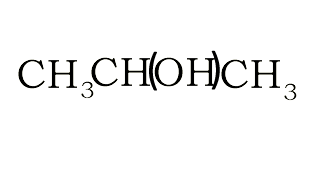Structural and semi-structural formulae The structural formula suggest a way in which the atoms of a molecule are connected. A structural formula may reveal the type of bond that exists between atoms and the bond angle. In our examples below, we have not revealed the bond angles but rather simplified the structural formulae. The semi-strucutural formula shows the order in which the atoms or group of atoms are connected in a molecule. Consider the compound with molecular formula C4H8O2. The molecule can have a number of possible isomers. Isomers are molecules with the same molecular formula but different structural formula. The structural formula allows us to differentiate between the two isomers. Consider the structural and semi-structural formulae of two isomers given below. The molecular formula alone is not enough to allow us to distinguish between the two forms, but given the structural and semi-structural formulae the distinction is clear.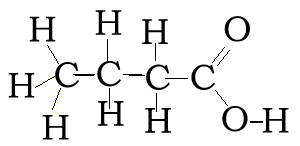CH3CH2CH2COOH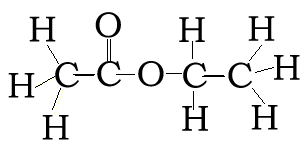CH3COOCH2CH3 Complete the table below. Click on the solutions link for extra assistance.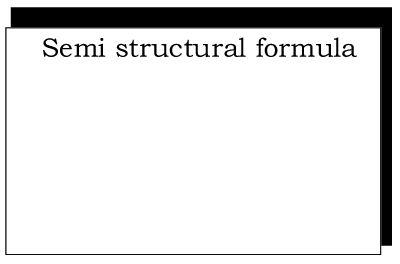Solution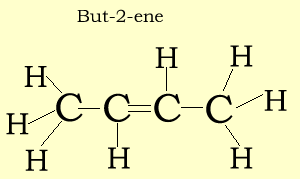Solution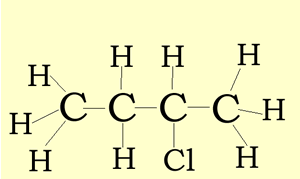Solution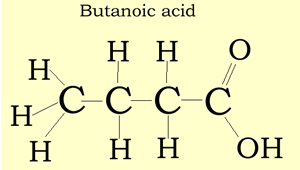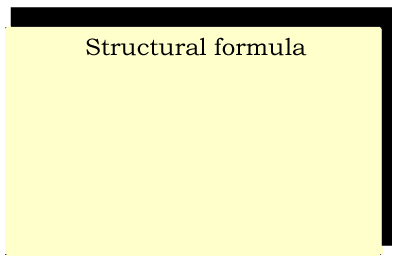Solution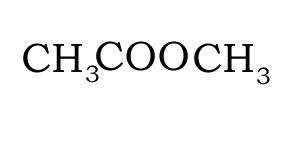Solution